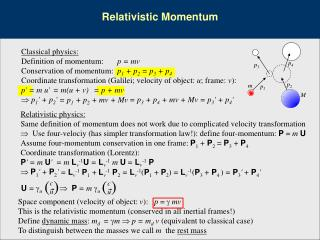DownloadDownload PresentationRelativistic Momentum

Relativistic Momentum

Download PresentationRelativistic Momentum

- - - - - - - - - - - - - - - - - - - - - - - - - - - E N D - - - - - - - - - - - - - - - - - - - - - - - - - - -
Presentation Transcript

1. Relativistic Momentum Classical physics: Definition of momentum: p = mv Conservation of momentum: p1 + p2 = p3 + p4 Coordinate transformation (Galilei; velocity of object: u; frame: v): p' = m u' p1' + p2' p4 p3 p2 m p1 = m(u + v) = p + mv M = p3 + p4 + mv + Mv = p3' + p4' = p1 + p2 + mv + Mv Relativistic physics: Same definition of momentum does not work due to complicated velocity transformation  Use four-velociy (has simpler transformation law!): define four-momentum:P = m U Assume four-momentum conservation in one frame: P1 + P2 = P3 + P4 Coordinate transformation (Lorentz): P' = m U' P1' + P2' = m Lv-1U = Lv-1 m U = Lv-1P = Lv-1P1+Lv-1P2 = Lv-1(P1+P2) = Lv-1(P3 + P4 ) = P3' + P4' ( ) ( ) Space component (velocity of object: v): p =mv This is the relativistic momentum (conserved in all inertial frames!) Define dynamic mass: md = m  p = md v (equivalent to classical case) To distinguish between the masses we call m the rest mass c u c u U = u  P = m u

2. Relativistic Momentum and Energy energy c 1 v2 2 c2 1 1 c 2 mc 1 – v2/c2 Time component : mc = – (mc2 +– mv2) = ––––––– = ––––––– ≈ mc (1 + – –– ) Time component of four-momentum conservation (same approximation, v << c ): (mc2 + ½ mv12) + (Mc2 + ½ Mv22 ) = (mc2 + ½ mv32) + (Mc2 + ½ Mv42 ) E1 + E2 = E3 + E4 Conservation of Energy! Total energy: E = mc2 Rest energy: E0 = mc2 (Energy for v = 0) Kinetic energy: Ekin = E – E0 =  mc2 – mc2 = (g – 1) mc2 Some important relations Analog to the spacetime interval we calculate the ‘magnitude’ of the four-momentum: P2 = (E/c)2 – p2 = For m  0 (p2c2 >> m2c4): Velocity in terms of E and p : = md c2 (mc)2 – (mv)2 = 2m2c2(1 – v2/c2) = m2c2  E2 = p2c2 + m2c4 E = pc v = p/(m) = pc2/E ; for m  0 : v = c Coordinate transformation: P = m U = mLvU' = LvP'  E' = g (E – v px); px' = g (px – v/c2 E); py' = py; pz' = pz

3. p q px v M L M L Relativistic Momentum and Energy 1 – v/c 1 – v2/c2 1 – v/c 1 + v/c = E––––––– = E–––– Energy transformation and Doppler shift Energy transformation E' = g (E – v px) Identical to Doppler shift formulas  Energy proportional to frequency (for m = 0)  Can be used to easily deduce general formula for Doppler shift: E' = g (E – v px)  fE= fRg(1 – v/ccosq) for massless objects ( p = E/c;assume p = px)  = g (E – v E/c) For px = 0: E' = gE = g (E – v pcosq) = Eg (1 – v/ccosq) (for m = 0) px = p cos q Equivalence of Mass and Energy: Einstein’s box Centre of mass cannot shift in isolated system  light pulse has a mass equivalent: Conservation of momentum: p = E/c = Mv Total time of process: t = L/c CM shift of box: MDx= Mvt = pL/c = E/c2L CM shift of light: mlightDxlight = mlightL  mlight = E/c2 Dx

4. Headlight Effect Laboratory frame E-M Radiation emitted isotropically Rest frame of emitter v = 0.9 c v = 0.9 c Galilean transf. v = 0.99 c

5. at rest in S at rest in S' S' S I S' – Q Relativistic Wire charged wire uncharged wire I S B = µI/2d B = µI/2d v FB = – Qv×B FB = – Qv×B = 0 – Q Electrostatic force (attractive) Lorentz force (attractive)

6. General Relativity: Spacetime curvature

7. General Relativity: Spacetime curvature

8. General Relativity: Gravitational Lensing

9. ct Future y Elsewhere x Past General Relativity: Spacetime Diagram, Light Cones ct' General Relativity x' falling into Special Relativity Object …. Black hole